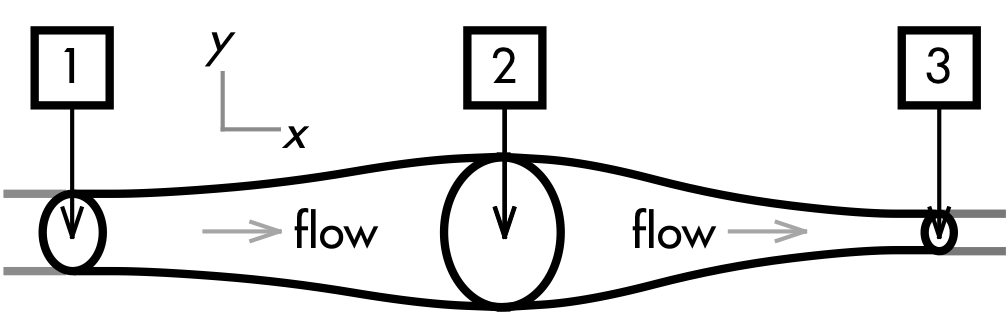## 20191104

### Physics quiz question: horizontal pipe with varying cross-sectional areas

Physics 205A Quiz 5, fall semester 2019
Cuesta College, San Luis Obispo, CA

Assume ideal fluid flow for water through this horizontal pipe with different cross-sectional areas.The greatest pressure is at:
(A) point .
(B) point .
(C) point .
(D) (There is a tie.)

Correct answer (highlight to unhide): (B)

From applying the continuity equation:

A1·v1 = A2·v2 = A3·v3,

where the fluid volume flow rate is the same throughout each of these three sections of pipe. As the cross-sectional area of the pipe is smallest at point  and largest at point , then:

A3 < A1 < A2,

such that the speeds at each section of pipe can be ordered accordingly, where the fastest speed occurs where the cross-sectional area is the narrowest:

v2 < v1 < v3.

Then from Bernoulli's equation:

0 = ∆P + (1/2)·ρ·∆(v2) + ρ·g·∆y,

because the pipe is horizontal, then ∆y = 0, and we can neglect the last term, such that:

0 = ∆P + (1/2)·ρ·∆(v2).

Comparing points  and  gives us:

0 = P2P1 + (1/2)·ρ·((v2)2 – (v1)2),

P1 + (1/2)·ρ·(v1)2 = P2 + (1/2)·ρ·(v2)2,

and similarly comparing points  and  gives us:

0 = P3P2 + (1/2)·ρ·((v3)2 – (v2)2),

P2 + (1/2)·ρ·(v2)2 = P3 + (1/2)·ρ·(v3)2.

Thus we can now compare the pressure and (1/2)·ρ·v2 terms for all three points:

P1 + (1/2)·ρ·(v1)2 = P2 + (1/2)·ρ·(v2)2 = P3 + (1/2)·ρ·(v3)2,

where the location with the smallest (1/2)·ρ·v2 term would correspond to having the greatest pressure. Earlier, from the continuity equation, since v2 < v1 < v3, then:

P2 > P1 > P3,

such that location  (having the largest area and slowest speed) would have the greatest pressure.

Sections 70854, 70855
Exam code: quiz05Gu1L
(A) : 1 student
(B) : 35 students
(C) : 10 students
(D) : 6 students

Success level: 67%
Discrimination index (Aubrecht & Aubrecht, 1983): 0.66